Derivatives in parametric form

Chapter 5 Class 12 Continuity and Differentiability
Concept wise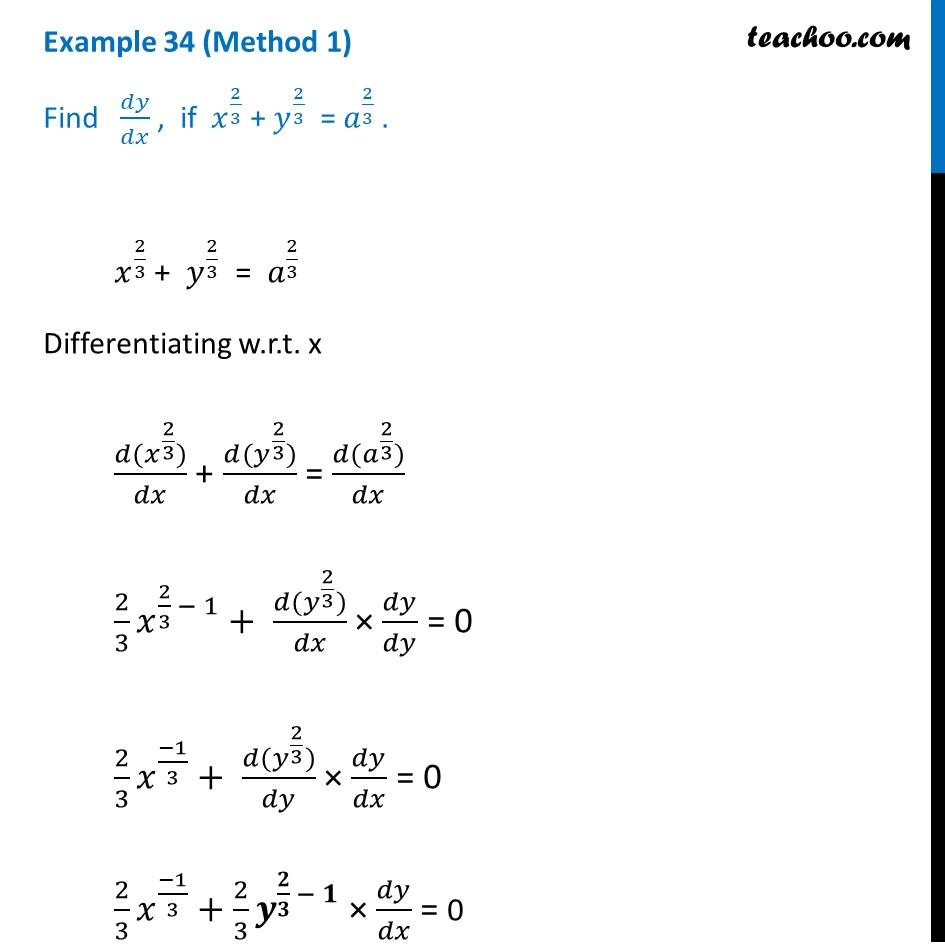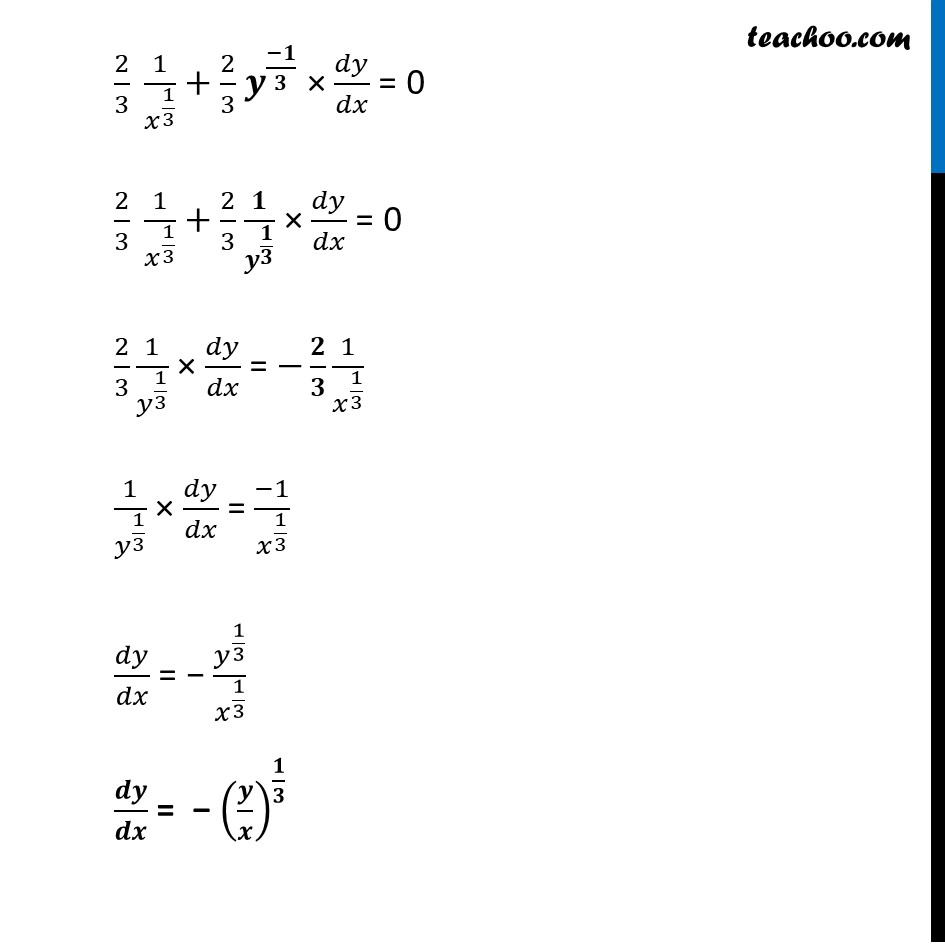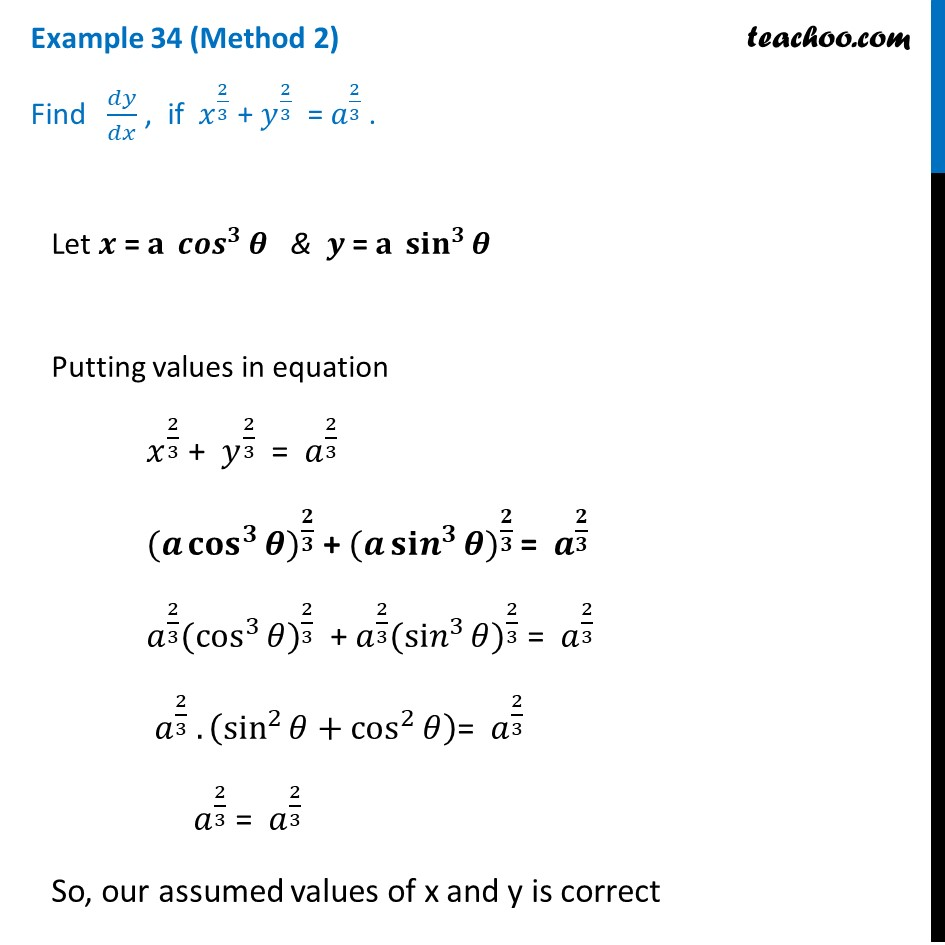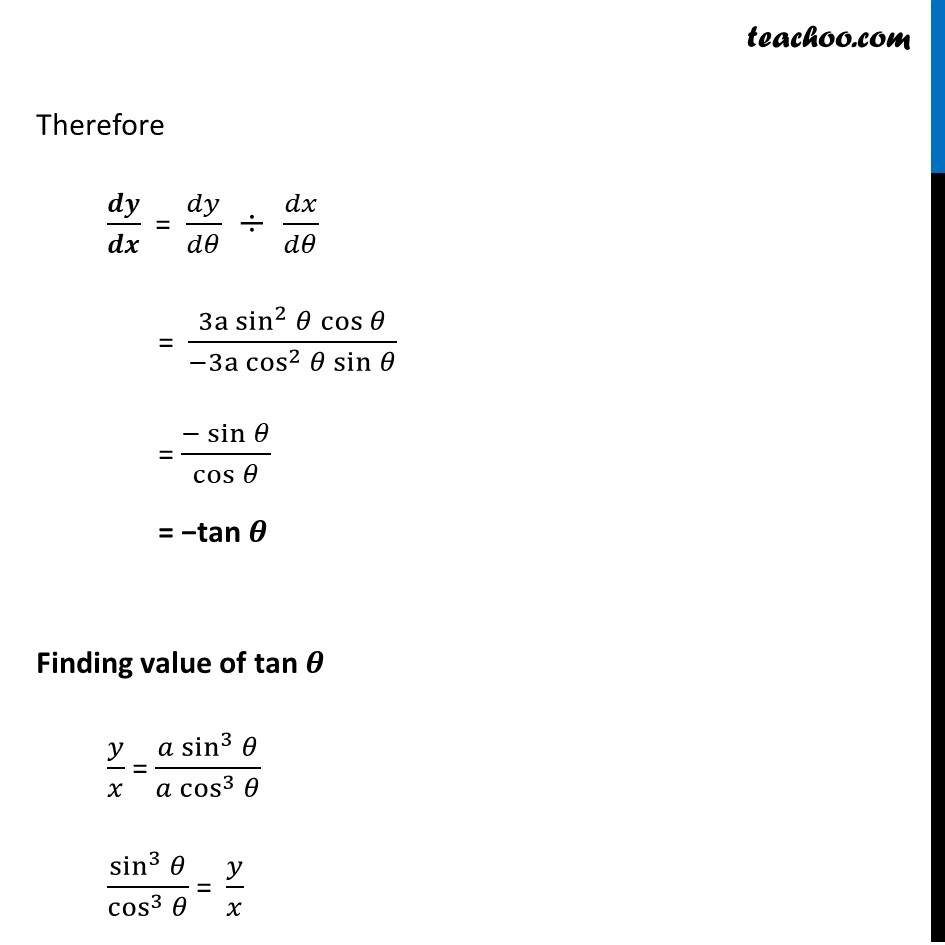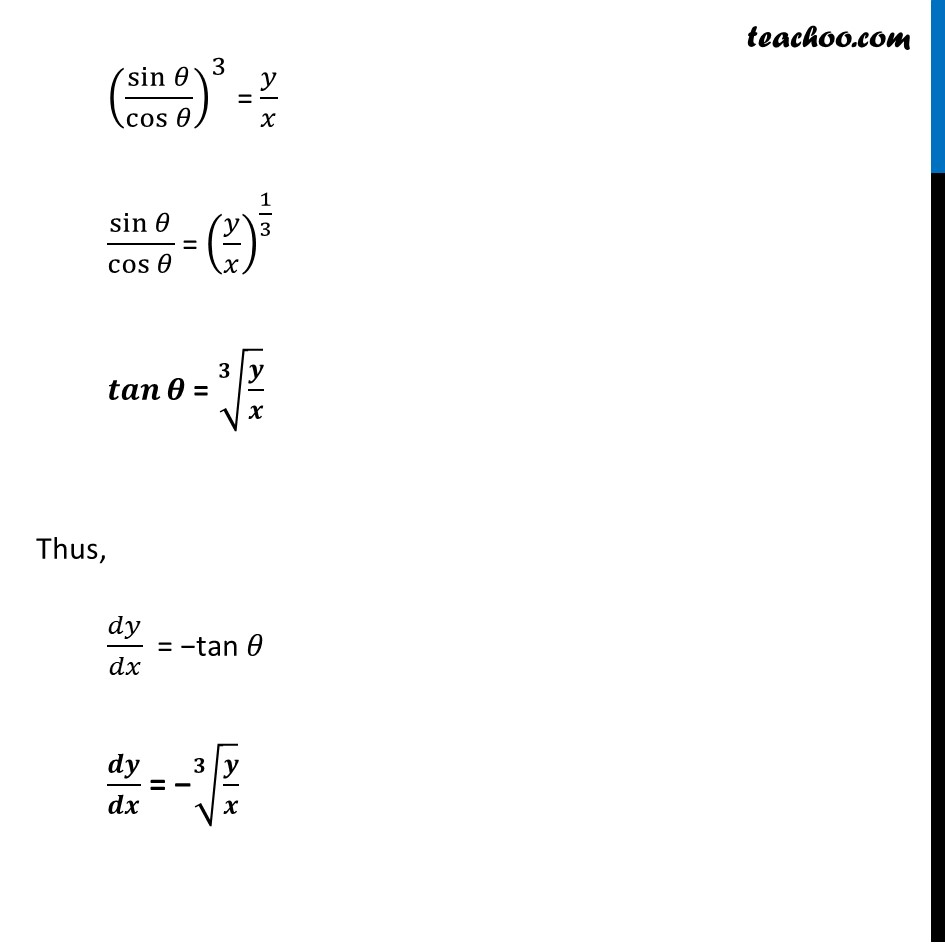Learn in your speed, with individual attention - Teachoo Maths 1-on-1 Class

### Transcript

Example 34 (Method 1) Find 𝑑𝑦/𝑑𝑥 , if 𝑥^(2/3) + 𝑦^(2/3) = 𝑎^(2/3) . 𝑥^(2/3) + 𝑦^(2/3) = 𝑎^(2/3) Differentiating w.r.t. x (𝑑(𝑥^(2/3)))/𝑑𝑥 + (𝑑(𝑦^(2/3)))/𝑑𝑥 = (𝑑(𝑎^(2/3)))/𝑑𝑥 2/3 𝑥^(2/3 − 1) + (𝑑(𝑦^(2/3)))/𝑑𝑥 × 𝑑𝑦/𝑑𝑦 = 0 2/3 𝑥^((−1)/3) + (𝑑(𝑦^(2/3)))/𝑑𝑦 × 𝑑𝑦/𝑑𝑥 = 0 2/3 𝑥^((−1)/3) + 2/3 𝒚^(𝟐/𝟑 − 𝟏) × 𝑑𝑦/𝑑𝑥 = 0 2/3 1/𝑥^(1/3) + 2/3 𝒚^((−𝟏)/𝟑) × 𝑑𝑦/𝑑𝑥 = 0 2/3 1/𝑥^(1/3) + 2/3 𝟏/𝒚^(𝟏/𝟑) × 𝑑𝑦/𝑑𝑥 = 0 2/3 1/𝑦^(1/3) × 𝑑𝑦/𝑑𝑥 = −𝟐/𝟑 1/𝑥^(1/3) 1/𝑦^(1/3) × 𝑑𝑦/𝑑𝑥 = (−1)/𝑥^(1/3) 𝑑𝑦/𝑑𝑥 = − 𝑦^(1/3)/𝑥^(1/3) 𝒅𝒚/𝒅𝒙 = − (𝒚/𝒙)^(𝟏/𝟑) Example 34 (Method 2) Find 𝑑𝑦/𝑑𝑥 , if 𝑥^(2/3) + 𝑦^(2/3) = 𝑎^(2/3) . Let 𝒙 = 𝐚 〖𝒄𝒐𝒔〗^𝟑⁡𝜽 & 𝒚 = 𝐚 〖𝐬𝐢𝐧〗^𝟑⁡𝜽 Putting values in equation 𝑥^(2/3) + 𝑦^(2/3) = 𝑎^(2/3) 〖(𝒂 〖𝐜𝐨𝐬〗^𝟑⁡𝜽)〗^(𝟐/𝟑) + 〖(𝒂 〖𝐬𝐢𝒏〗^𝟑⁡𝜽)〗^(𝟐/𝟑) = 𝒂^(𝟐/𝟑) 𝑎^(2/3) 〖(cos^3⁡𝜃)〗^(2/3) + 𝑎^(2/3) 〖(〖si𝑛〗^3⁡𝜃)〗^(2/3) = 𝑎^(2/3) 𝑎^(2/3) . (sin^2⁡𝜃+cos^2⁡𝜃 )= 𝑎^(2/3) 𝑎^(2/3) = 𝑎^(2/3) So, our assumed values of x and y is correct Now, 𝑑𝑦/𝑑𝑥 = (𝑑𝑦/𝑑𝜃)/(𝑑𝑥/𝑑𝜃) Calculating 𝒅𝒚/𝒅𝜽 𝑦 = a sin^3⁡𝜃 𝑑𝑦/𝑑𝜃 = 𝑑(a sin^3⁡𝜃 )/𝑑𝜃 𝑑𝑦/𝑑𝜃 = a (3 sin^2⁡𝜃) 𝒅(𝒔𝒊𝒏⁡𝜽 )/𝒅𝜽 𝑑𝑦/𝑑𝜃 = 3𝑎 sin2 𝜽 cos 𝜽 Calculating 𝒅𝒙/𝒅𝜽 𝑥 = a cos^3⁡𝜃 𝑑𝑥/𝑑𝜃 = (𝑑 (〖a cos〗^3⁡〖𝜃)〗)/𝑑𝜃 𝑑𝑥/𝑑𝜃 = a(3 cos^2⁡𝜃) (𝒅(𝒄𝒐𝒔 𝜽) )/𝒅𝜽 𝒅𝒙/𝒅𝜽 = 𝟑𝐚 〖𝒄𝒐𝒔〗^𝟐⁡𝜽 . (−𝒔𝒊𝒏⁡𝜽 ) Therefore 𝒅𝒚/𝒅𝒙 = 𝑑𝑦/𝑑𝜃 ÷ 𝑑𝑥/𝑑𝜃 = (3a sin^2⁡𝜃 cos⁡𝜃)/(−3a cos^2⁡𝜃 sin⁡𝜃 ) = (−sin⁡𝜃)/cos⁡𝜃 = −tan 𝜽 Finding value of tan 𝜽 𝑦/𝑥 = (𝑎 sin^3⁡𝜃)/(𝑎 cos^3⁡𝜃 ) sin^3⁡𝜃/cos^3⁡𝜃 = 𝑦/𝑥 (sin⁡𝜃/cos⁡𝜃 )^3 = 𝑦/𝑥 sin⁡𝜃/cos⁡𝜃 = (𝑦/𝑥)^(1/3) 𝒕𝒂𝒏⁡𝜽 = √(𝟑&𝒚/𝒙) Thus, 𝑑𝑦/𝑑𝑥 = −tan 𝜃 𝒅𝒚/𝒅𝒙 = −√(𝟑&𝒚/𝒙)